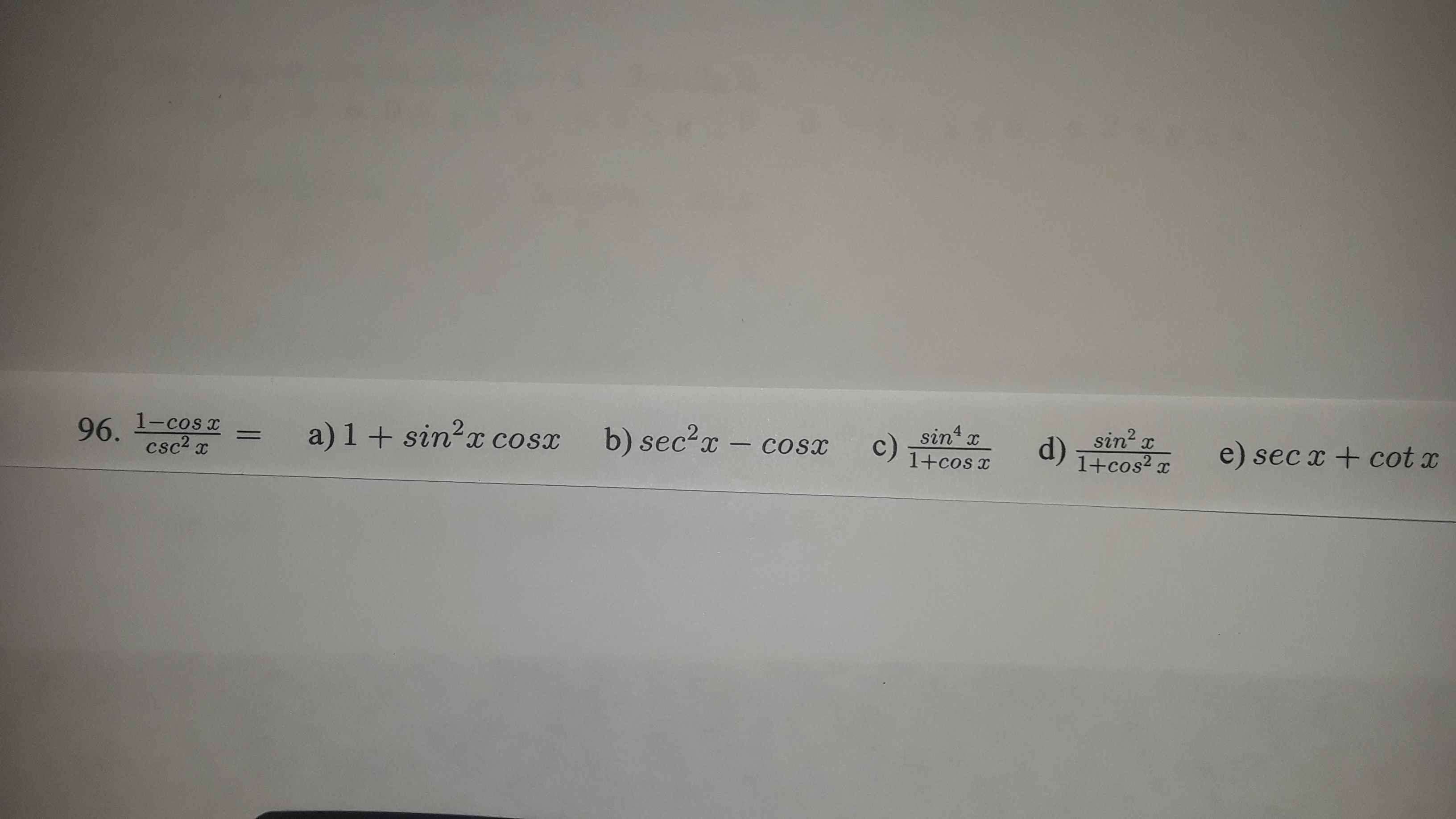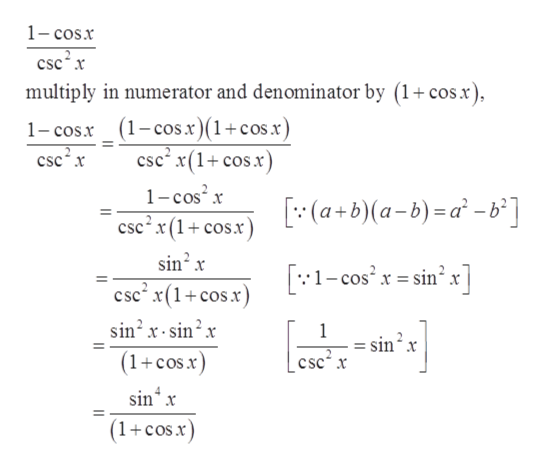# sin x1-cos x96.csc2 xa) 1+ sin?x cosxb) sec?xc)d)sin? x1+cos? xe) sec x + cot x%3DCosx1+cos x

Question
1 viewshelp_outlineImage Transcriptionclosesin x 1-cos x 96. csc2 x a) 1+ sin?x cosx b) sec?x c) d) sin? x 1+cos? x e) sec x + cot x %3D Cosx 1+cos x fullscreen
check_circle

Step 1

Given:

Step 2

Expand the given trigono...help_outlineImage Transcriptionclose1- cos.x csc x multiply in numerator and denominator by (1+cos.x), 1- cos.x_ (1-cos.x)(1+cosx) csc²x csc x(1+ cos.x) 1-cos x [:(a+b)(a-b)=a° - °] csc? x (1+ cos.x) sin? x [:1-cos" x = sin x] csc x(1+cos.x) sin'x- sin?x (1+cos.x) sin² x csc x sin“ x (1+cosx) fullscreen

### Want to see the full answer?

See Solution

#### Want to see this answer and more?

Solutions are written by subject experts who are available 24/7. Questions are typically answered within 1 hour.*

See Solution
*Response times may vary by subject and question.
Tagged in
MathTrigonometry

### Trigonometric Ratios